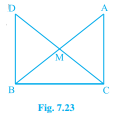# In right triangle $\mathrm{ABC}$, right angled at $\mathrm{C}, \mathrm{M}$ is the mid-point of hypotenuse $\mathrm{AB}$. $\mathrm{C}$ is joined to $\mathrm{M}$ and produced to a point $\mathrm{D}$ such that $\mathrm{DM}=\mathrm{CM}$. Point $\mathrm{D}$ is joined to point $\mathrm{B}$ (see Fig. 7.23). Show that:(i) $\triangle \mathrm{AMC} \equiv \triangle \mathrm{BMD}$(ii) $\angle \mathrm{DBC}$ is a right angle.(iii) $\triangle \mathrm{DBC} \equiv \triangle \mathrm{ACB}$(iv) $\mathrm{CM}=\frac{1}{2} \mathrm{AB}$"

Given:

In right triangle $ABC$, right angled at $C$, $M$ is the mid-point of hypotenuse $AB$. $C$ is joined to $M$ and produced to a point $D$ such that  $DM=CM$. Point $D$ is joined to point $B$.

To do:

We have to show the given questions.

Solution:

(i) Let us consider $\triangle AMC$ and $\triangle BMD$,

We know that,

According to Rule of Side-Angle-Side Congruence:

Triangles are said to be congruent if any pair of corresponding sides and their included angles are equal in both triangles.

Given,

$CM=DM$ and

$M$ is the midpoint of the hypotenuse $AB$

This implies,

$AM=BM$

We also know that,

The vertically opposite angles are always equal,

This implies,

$\angle CMA=\angle DMB$

Therefore,

By SAS congruence,

$\triangle AMC \cong \triangle BMD$.

(ii) We also know,

From corresponding parts of congruent triangles: If two triangles are congruent, all of their corresponding angles and sides  must be equal.

This implies,

$\angle ACM=\angle BDM$

We know that,

if the alternate interior angles were equal, then the two lines were parallel.

This implies,

$AC \parallel BD$

We also know that, the sum of the interior angles is equal to $180^o$

This implies,

$\angle ACB+\angle DBC=180^o$

$90^o+\angle DBC=180^o$   (given, $\triangle ABC$ is right angled at $c$)

This implies,

$\angle DBC=90^o$

(iii) Let us consider $\triangle DBC$ and $\triangle ACB$

We know that,

According to Rule of Side-Angle-Side Congruence:

Triangles are said to be congruent if any pair of corresponding sides and their included angles are equal in both triangles.

$BC=CB$ (common side)

Since,$\angle ACB$ and $DBC$ are right angles to each other we get,

$\angle ACB=\angle DBC$

We also know,

From corresponding parts of congruent triangles: If two triangles are congruent, all of their corresponding  sides must be equal.

Therefore,

$DB=AC$.

Therefore,

$\triangle DBC \cong ACB$.

(iv) Since $\triangle DBC \cong ACB$

We get,

$DM=AB$

We also have $M$ as the mid point

This implies,

$DM=CM=AM=BM$

Therefore,

$DM+CM=BM+AM$

$CM+CM=AB$

This implies,

$CM=\frac{1}{2}AB$.